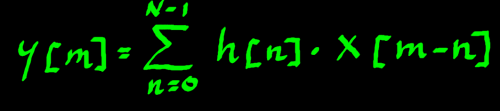# Convolution

A Matrix Operation

 From "Wavelets and Filter Banks" by Gilbert Strang and Truong Nguyen, I learnt that convolution can be considered a matrix operation. Convolution can do (no more than) amplification and attenuation of frequencies by means of phase cancellation/reinforcement. The popular name is therefore: filtering.In convolution, three arrays of sample values appear:
an input array x[n]
an impuls response array h[n] (the 'filter')
an output array y[n]

[n] denotes a sample index number. The index can be related to time or space if you want. The impuls response h[n] is responsible for the amplification / attenuation. For convenience I will call that array 'filter'. Only later on this page I can illustrate what it means that the filter is an impulse response.

Below is the simplest of convolutions as an example to start with. A one-sample 'filter' h is on the main diagonal, which is also called identity diagonal. The input array and output array are vectors of equal length here. They could be streams of audio with a million or a billion of samples as well. That would make a very big square filter matrix, but still with only one diagonal.Such a convolution with h can only amplify or atttenuate all frequencies at the same time. Not what we call a filter yet.

Let us next do an example with a two-sample h[n]. That can already make a useful filter in practice. If you inspect the rows of the filter below, you will notice that the array h[n] appears time-reversed in the matrix. That is a major aspect of convolution.

As compared to the one-sample filter, an extra row in the filter matrix, and thus in the output, is required to complete the convolution with the two-sample filter.One more example to see the system of it. The diagonals are constant. All filter coefficients h[n] have a fair share in the operation. They appear as many times as there are input samples. There is also a rule of thumb for the output array-length:

N[filter] + N[input] -1 = N[output]

In the example below it is: ((3+4)-1) = 6Below, the correlation for output index y is illustrated as an example. The rows of the filter matrix are numbered, and they correspond to the output rows. Notice that x is multiplied with the filter's identity diagonal. Furthermore, some older samples of x[n] are used.Fortunately webpixels cost nothing so I can afford doing lots of pictures. Below is the correlation for y. It is almost hard to distinguish it from the preceding picture, because the operation is so systematic. So let us try to identify the pattern of it.Admittedly it took me quite some time to disclose the pattern in a sensible way. But look, the index number of x[n] can be described in terms of the y[n] and h[n] indices.In order to write the whole thing in an algebraic formula, some index letters will have to be renamed, unfortunately. I suggest that we use x[m], y[m] and h[n]. Then the whole convolution can be described efficiently in terms of the arrays:Suppose the filter array is a fixed buffer and the input array is a stream. Then it would be effective to keep older values of x[n] in a circular buffer or (multitap) delayline. That is indeed how filters of modest length are done. Below is the flowchart of the example filter.One question is still not answered: why is h[n] called 'impulse response'? Again, this can be illustrated nicely with a simple matrix (I love them). For the input array x[n], we choose a unit puls or impulse as it is called. In the digital domain that means: a samplevalue of 1 on x and zero's everywhere else. Now look at the output (the response to the impulse). It is the filter array itself! So at the ouput it appears in the normal time-direction....The filter matrix implements reflections. The later reflections (higher index numbers of h[n]) have a longer delay time. That is why h[n] appears time-reversed in the matrix operation. The higher h[n] indices must operate on older samples of x[n]. And older samples means: lower index numbers.

There is a strong analogy with acoustic reflections. Imagine you do a pulse in a reflective space, by clapping your hands once. The response to that pulse is: the direct sound h, the first reflection from a nearby surface h, and the second reflection from a surface further away h. These reflections will also do phase cancellation and reinforcement, thus filtering.The impulse response is a soundwise blueprint of a space or system. Once you have captured it digitally, or computed an idealised impulse response, you have your filter array.